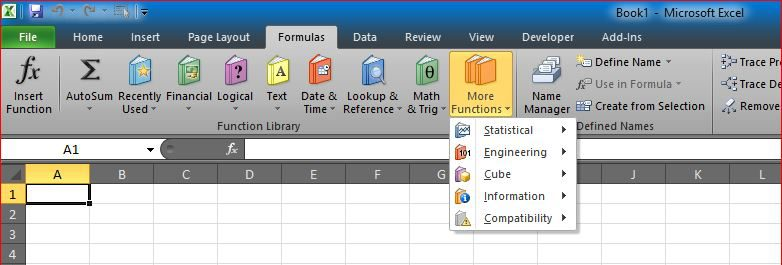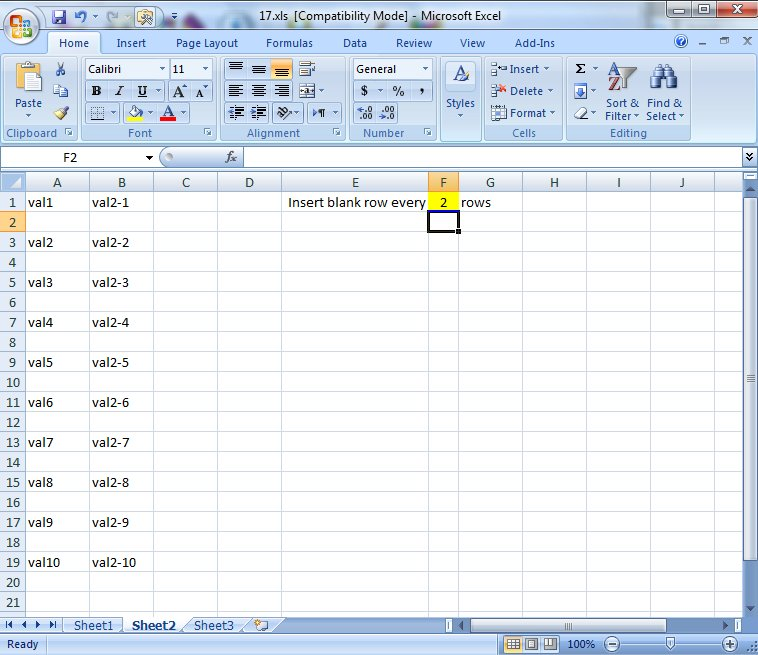# Insert Blank rows into table using functionsHere you will see how to work with functions to insert a blank row every certain number of rows in a table using a blank sheet to copy all values of that table into the new sheet
Then using Copy > Paste Special to make them constants
The new thing is that this is all done using only functions (Except the Copy and Paste for sure)

Formula used:

`=IF(ROW()/\$F\$1=INT(ROW()/\$F\$1),"",INDIRECT("Sheet1!"&CHAR(COLUMN()+64)&INT(ROW()/\$F\$1)-(INT(ROW()/\$F\$1))*2+ROW()))`